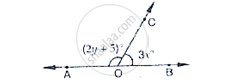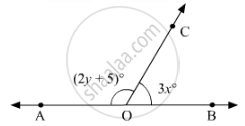# In the Below Fig, Oa and Ob Are Opposite Rays If X = 25°, What is the Value of Y? - Mathematics

In the below Fig, OA and OB are opposite rays :

If x = 25°, what is the value of y?#### Solution 1

Given that x = 25°

Since ∠AOC and ∠BOC form a linear pair

∠AOC + ∠BOC = 180°

Given that

∠AOC = 2 y + 5 and ∠BOC = 3x

∴ ∠AOC + ∠BOC = 180°

(2 y + 5)° + 3x = 180°

(2 y + 5)° + 3(25°) = 180°

2 y° + 5° + 75° = 180°

2 y° + 80° = 180°

2 y° = 180° - 80° = 100°

y° = (100°)/2 = 50°

⇒  y = 50°

#### Solution 2

In figure:

Since OA and OB are opposite rays. Therefore, AB is a line. Since, OC stands on line AB.

Thus,∠AOCand ∠BOC form a linear pair, therefore, their sum must be equal to180°.Or, we can say that

∠AOC + ∠BOC = 180°

From the given figure:

∠AOC= (2y + 5)and ∠BOC = 3x

On substituting these two values, we get

(2y + 5) + 3x = 180

3x + 2y = 180 -5

3x + 2y = 175                            ...(i)

On putting x = 25in (i), we get:

3(25 )+2y = 175

75 + 2y = 175

2y = 175 - 75

2y = 100

y = 100/2

y = 50

Hence, the value of y is 50.

Concept: Pairs of Angles
Is there an error in this question or solution?

#### APPEARS IN

RD Sharma Mathematics for Class 9
Chapter 10 Lines and Angles
Exercise 10.2 | Q 1.1 | Page 14